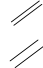### 2.5.3 New markup command definition

This section discusses the definition of new markup commands.

#### Markup command definition syntax

New markup commands can be defined using the `define-markup-command` Scheme macro, at top-level.

 ```(define-markup-command (command-name layout props arg1 arg2 …) (arg1-type? arg2-type? …) [ #:properties ((property1 default-value1) …) ] …command body…) ```

The arguments are

`command-name`

the markup command name

`layout`

the ‘layout’ definition.

`props`

a list of associative lists, containing all active properties.

`argi`

ith command argument

`argi-type?`

a type predicate for the ith argument

If the command uses properties from the `props` arguments, the `#:properties` keyword can be used to specify which properties are used along with their default values.

Arguments are distinguished according to their type:

• a markup, corresponding to type predicate `markup?`;
• a list of markups, corresponding to type predicate `markup-list?`;
• any other scheme object, corresponding to type predicates such as `list?`, `number?`, `boolean?`, etc.

There is no limitation on the order of arguments (after the standard `layout` and `props` arguments). However, markup functions taking a markup as their last argument are somewhat special as you can apply them to a markup list, and the result is a markup list where the markup function (with the specified leading arguments) has been applied to every element of the original markup list.

Since replicating the leading arguments for applying a markup function to a markup list is cheap mostly for Scheme arguments, you avoid performance pitfalls by just using Scheme arguments for the leading arguments of markup functions that take a markup as their last argument.

Markup commands have a rather complex life cycle. The body of a markup command definition is responsible for converting the arguments of the markup command into a stencil expression which is returned. Quite often this is accomplished by calling the `interpret-markup` function on a markup expression, passing the layout and props arguments on to it. Those arguments are usually only known at a very late stage in typesetting. Markup expressions have their components assembled into markup expressions already when `\markup` in a LilyPond expression or the `markup` macro in Scheme is expanded. The evaluation and typechecking of markup command arguments happens at the time `\markup`/`markup` are interpreted.

But the actual conversion of markup expressions into stencil expressions by executing the markup function bodies only happens when `interpret-markup` is called on a markup expression.

#### On properties

The `layout` and `props` arguments of markup commands bring a context for the markup interpretation: font size, line width, etc.

The `layout` argument allows access to properties defined in `paper` blocks, using the `ly:output-def-lookup` function. For instance, the line width (the same as the one used in scores) is read using:

```(ly:output-def-lookup layout 'line-width)
```

The `props` argument makes some properties accessible to markup commands. For instance, when a book title markup is interpreted, all the variables defined in the `\header` block are automatically added to `props`, so that the book title markup can access the book title, composer, etc. It is also a way to configure the behaviour of a markup command: for example, when a command uses font size during processing, the font size is read from `props` rather than having a `font-size` argument. The caller of a markup command may change the value of the font size property in order to change the behaviour. Use the `#:properties` keyword of `define-markup-command` to specify which properties shall be read from the `props` arguments.

The example in next section illustrates how to access and override properties in a markup command.

#### A complete example

The following example defines a markup command to draw a double box around a piece of text.

Firstly, we need to build an approximative result using markups. Consulting the Text markup commands shows us the `\box` command is useful:

```\markup \box \box HELLO
```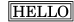Now, we consider that more padding between the text and the boxes is preferable. According to the `\box` documentation, this command uses a `box-padding` property, which defaults to 0.2. The documentation also mentions how to override it:

```\markup \box \override #'(box-padding . 0.6) \box A
```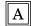Then, the padding between the two boxes is considered too small, so we override it too:

```\markup \override #'(box-padding . 0.4) \box
\override #'(box-padding . 0.6) \box A
```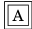Repeating this lengthy markup would be painful. This is where a markup command is needed. Thus, we write a `double-box` markup command, taking one argument (the text). This draws the two boxes, with some padding.

 ```#(define-markup-command (double-box layout props text) (markup?) "Draw a double box around text." (interpret-markup layout props #{\markup \override #'(box-padding . 0.4) \box \override #'(box-padding . 0.6) \box { #text }#})) ```

or, equivalently

 ```#(define-markup-command (double-box layout props text) (markup?) "Draw a double box around text." (interpret-markup layout props (markup #:override '(box-padding . 0.4) #:box #:override '(box-padding . 0.6) #:box text))) ```

`text` is the name of the command argument, and `markup?` its type: it identifies it as a markup. The `interpret-markup` function is used in most of markup commands: it builds a stencil, using `layout`, `props`, and a markup. In the second case, this markup is built using the `markup` scheme macro, see Markup construction in Scheme. The transformation from `\markup` expression to scheme markup expression is straight-forward.

The new command can be used as follow:

```\markup \double-box A
```

It would be nice to make the `double-box` command customizable: here, the `box-padding` values are hard coded, and cannot be changed by the user. Also, it would be better to distinguish the padding between the two boxes, from the padding between the inner box and the text. So we will introduce a new property, `inter-box-padding`, for the padding between the two boxes. The `box-padding` will be used for the inner padding. The new code is now as follows:

 ```#(define-markup-command (double-box layout props text) (markup?) #:properties ((inter-box-padding 0.4) (box-padding 0.6)) "Draw a double box around text." (interpret-markup layout props #{\markup \override #`(box-padding . ,inter-box-padding) \box \override #`(box-padding . ,box-padding) \box { #text } #})) ```

Again, the equivalent version using the markup macro would be:

 ```#(define-markup-command (double-box layout props text) (markup?) #:properties ((inter-box-padding 0.4) (box-padding 0.6)) "Draw a double box around text." (interpret-markup layout props (markup #:override `(box-padding . ,inter-box-padding) #:box #:override `(box-padding . ,box-padding) #:box text))) ```

Here, the `#:properties` keyword is used so that the `inter-box-padding` and `box-padding` properties are read from the `props` argument, and default values are given to them if the properties are not defined.

Then, these values are used to override the `box-padding` properties used by the two `\box` commands. Note the backquote and the comma in the `\override` argument: they allow you to introduce a variable value into a literal expression.

Now, the command can be used in a markup, and the boxes padding be customized:

```#(define-markup-command (double-box layout props text) (markup?)
"Draw a double box around text."
(interpret-markup layout props
{ #text } #}))

\markup \double-box A
\markup \override #'(inter-box-padding . 0.8) \double-box A
\markup \override #'(box-padding . 1.0) \double-box A
```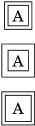A good way to start writing a new markup command, is to take example on a builtin one. Most of the markup commands provided with LilyPond can be found in file ‘scm/define-markup-commands.scm’.

For instance, we would like to adapt the `\draw-line` command, to draw a double line instead. The `\draw-line` command is defined as follow (documentation stripped):

 ```(define-markup-command (draw-line layout props dest) (number-pair?) #:category graphic #:properties ((thickness 1)) "…documentation…" (let ((th (* (ly:output-def-lookup layout 'line-thickness) thickness)) (x (car dest)) (y (cdr dest))) (make-line-stencil th 0 0 x y))) ```

To define a new command based on an existing one, copy the definition, and change the command name. The `#:category` keyword can be safely removed, as it is only used for generating LilyPond documentation, and is of no use for user-defined markup commands.

 ```(define-markup-command (draw-double-line layout props dest) (number-pair?) #:properties ((thickness 1)) "…documentation…" (let ((th (* (ly:output-def-lookup layout 'line-thickness) thickness)) (x (car dest)) (y (cdr dest))) (make-line-stencil th 0 0 x y))) ```

Then, a property for setting the gap between two lines is added, called `line-gap`, defaulting e.g. to 0.6:

 ```(define-markup-command (draw-double-line layout props dest) (number-pair?) #:properties ((thickness 1) (line-gap 0.6)) "…documentation…" … ```

Finally, the code for drawing two lines is added. Two calls to `make-line-stencil` are used to draw the lines, and the resulting stencils are combined using `ly:stencil-add`:

```#(define-markup-command (my-draw-line layout props dest)
(number-pair?)
#:properties ((thickness 1)
(line-gap 0.6))
"..documentation.."
(let* ((th (* (ly:output-def-lookup layout 'line-thickness)
thickness))
(dx (car dest))
(dy (cdr dest))
(w (/ line-gap 2.0))
(x (cond ((= dx 0) w)
((= dy 0) 0)
(else (/ w (sqrt (+ 1 (* (/ dx dy) (/ dx dy))))))))
(y (* (if (< (* dx dy) 0) 1 -1)
(cond ((= dy 0) w)
((= dx 0) 0)
(else (/ w (sqrt (+ 1 (* (/ dy dx) (/ dy dx))))))))))
(ly:stencil-add (make-line-stencil th x y (+ dx x) (+ dy y))
(make-line-stencil th (- x) (- y) (- dx x) (- dy y)))))

\markup \my-draw-line #'(4 . 3)
\markup \override #'(line-gap . 1.2) \my-draw-line #'(4 . 3)
```